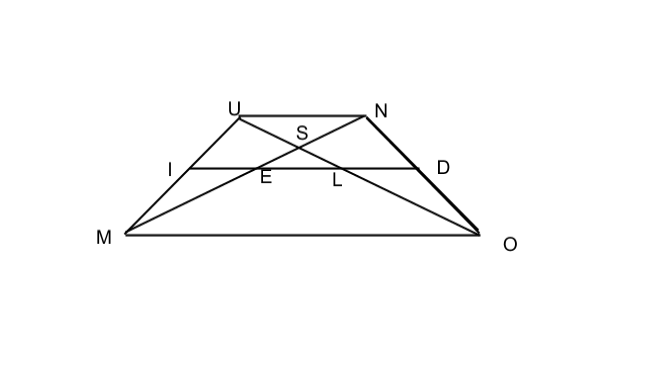Trapped Triangles

Geometry Level 3In the above figure, line segments UN, DI, and MO parallel, while I and D are the midpoints of UM and NO respectively. If MO = 64, UN = 28, and MN = 46, find ES.

×